# Binary Lennard-Jones mixtures

Jump to: navigation, searchThis article is a 'stub' page, it has no, or next to no, content. It is here at the moment to help form part of the structure of SklogWiki. If you add sufficient material to this article then please remove the {{Stub-general}} template from this page.

A mixture of Lennard-Jones sites

## Wahnström mixture

The Wahnström mixture is a equimolar composition of A and B particles with the Lennard-Jones parameters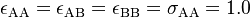$\epsilon_{\mathrm{AA}} = \epsilon_{\mathrm{AB}} = \epsilon_{\mathrm{BB}} = \sigma_{\mathrm{AA}} = 1.0$,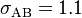$\sigma_{\mathrm{AB}} = 1.1$ and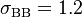$\sigma_{\mathrm{BB}} = 1.2$. At low temperature the liquid accumulate icosahedral order, and may partially crystallized into the MgZn2 crystal structure.

## Kob-Andersen mixture

The Kob-Andersen mixture    composed of 80% of atoms of type A, has the following parameters: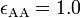$\epsilon_{\mathrm{AA}} = 1.0$,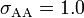$\sigma_{\mathrm{AA}} = 1.0$,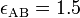$\epsilon_{\mathrm{AB}} = 1.5$,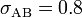$\sigma_{\mathrm{AB}} = 0.8$,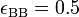$\epsilon_{\mathrm{BB}} = 0.5$ and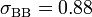$\sigma_{\mathrm{BB}} = 0.88$. The viscous dynamics of this mixture has been studied by Pedersen, Schrøder and Dyre . Glass transition . Phase diagram   .